# Chapter 5  Algorithm

#### AAA methodology

In the AAA methodology, an algorithm is specified as a directed acyclic graph (DAG) infinitely repeated. Directed means that for each edge representing a relation between vertices, the vertices tuple is ordered, i.e. its first element is the source vertex and the other one(s) is(are) the destination vertex(vertices). A vertex is an operation corresponding to a sequence of instructions which starts after all its input data are available and produces all its output data at the end of the sequence. An edge is a dependence between two vertices corresponding to a data transfer and an execution precedence, or to an execution precedence only. Note that some vertices may be independent, i.e. may not be connected by dependences.

#### Definition vs. reference

In SynDEx there is a distinction between algorithm definition and algorithm reference. A definition preexists to a reference that corresponds to one an only one definition. On the contrary, to a given definition may correspond several references. That allows for referencing, with different names, a unique definition. Therefore, an algorithm is described by a definition, which is a DAG similar to those in AAA, where vertices are references or ports, and edges are dependences between references, or between references and ports.

#### Atomic or hierarchical definitions

To a given reference contained in a definition corresponds a definition which may contain itself several references, and so on. That corresponds to hierarchy. A definition is said hierarchical when it defines an algorithm which contains at least one dependence connecting an input port to an output port, and possibly one or several references connected by dependences, otherwise it is said atomic.

There are five types of atomic definitions: functions read data on input ports, execute instructions without any side-effect, write data on output ports, sensors are input vertices of the DAG producing data from a physical sensor, actuators are output vertices of the DAG consuming data for a physical actuator, constants are input vertices of the DAG, with null execution time, delays memorize data during one or several infinite repetition of the DAG, for use in next repetitions. These types are detailed in section 5.1.1.

A definition is said explicitly hierarchical when the algorithm contains at least one dependence (and possibly references). This includes conditioning (cf. section 5.2), repetitions (cf. section 5.3) of hierarchical definitions, and more generally definitions defined through several levels of hierarchy. Only a function may be defined through explicit hierarchy.

A definition is said implicitly hierarchical when the algorithm does not contain any dependence and yet will be transformed by SynDEx, for the adequation, into a graph which contains dependences. This happens only with repetitions (cf. section 5.3) of atomic definitions.
Warning: A hierarchical definition does not have to wait for all its input data to be available before starting some computations. Indeed, parts of the algorithm graph of a hierarchical algorithm definition may only require parts of the input data of the definition and therefore can start as soon as this part is available (and not all the data). In the same way, some data may be produced before the end of the complete sequence of computations.

#### Dependences

There are two types of dependences:

• data dependence: data transfer and execution precedence,
• precedence dependence: execution precedence only.

A data dependence imposes that the reference at the source of the dependence, produces data and is executed before the reference at the destination of the dependence, which consumes the data. A precedence dependence only imposes an execution order between references, no data is produced or consumed.

#### Algorithm window

Definitions and references are managed through analgorithm window. If necessary it is possible to open several algorithm windows.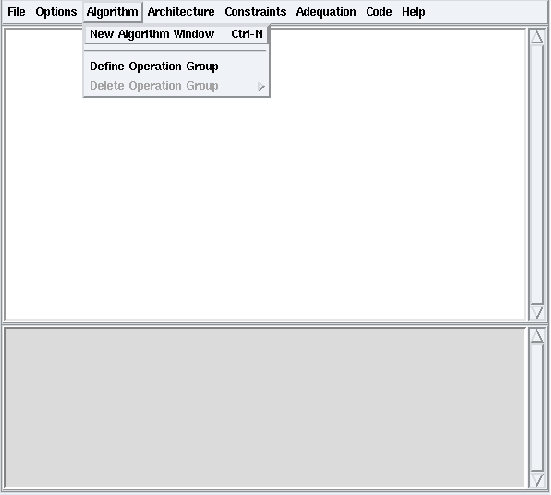Figure 5.1: Algorithm / New Algorithm Window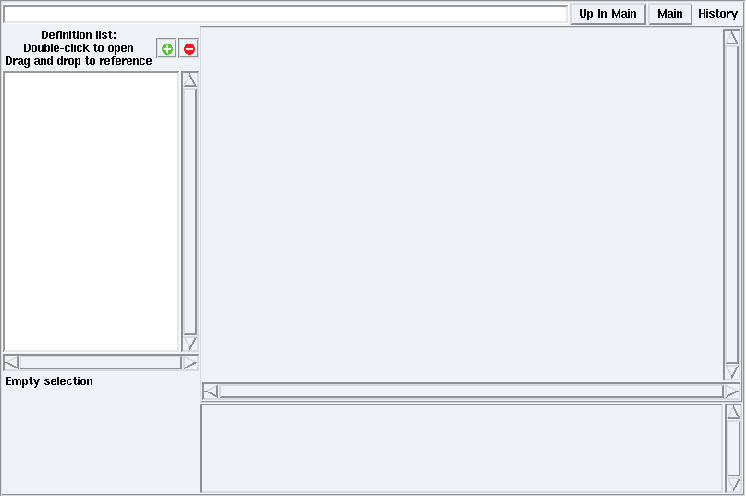Figure 5.2: Algorithm Window

From the Algorithm menu, choose the New Algorithm Window option (cf. figure 5.1). It opens an algorithm window for algorithm definitions (cf. figure 5.2). Left click on the background of a definition window: the algorithm window shows its Definition Properties. Left click on a reference in this definition window: the algorithm window shows its Reference Properties. These definition or reference properties appear in the left bottom part of the algorithm window (cf. figure 5.4 for definition properties and figure 5.5 for reference properties).

## 5.1  To create an algorithm definition

### 5.1.1  To create a definition

#### Types of definitions

SynDEx distinguishes five types of definitions with different edition rules:

• a function is a general abstraction with no edition restriction: it can contain dependences, references and ports;
• a sensor is an abstraction of a physical device producing data: it can only contain output ports;
• an actuator is an abstraction of a physical device consuming data: it can only contain input ports;
• a constant is a an abstraction of a typed value: it can only contain one output port producing that value. For convenience, the value hold by the constant can be given as a parameter to the constant definition. Note that this is only possible for values that are representable within the parameter language: integer, float, string and list of such values. SynDEx standard library uses this trick to define constants for the library base types (int, float, ...). For example, the cst definition of the int library has one parameter: ListOfValues;
• a delay is an abstraction of a memory region: it must contain one input port (the write port) and one output port (the read port) of the same type, but nothing more. Delays hold the state of a SynDEx application. Using delays is the only way to propagate data from one iteration of the application to the next. A delay must be initialized, either by using a parameter (as suggested above for constant definitions) or lately in the real world code (as for constant definitions, doing it in the code is the only alternative for delays holding values of complex types). SynDEx standard library defines delays for its base types as shift registers with two parameters: the first one is a list of initial values and the second one is the size (in number of elements) of the shift register. For example, the delay definition of the int library has two parameters: listInit and nbDelay.

#### New definition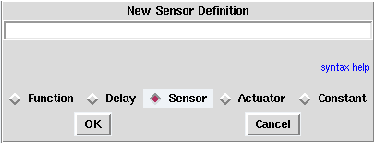Figure 5.3: Definition of a sensor

To create a new definition, in the algorithm window, left click on the + green button. It opens a dialog window in which you can select the definition’s type. For example check Sensor (cf. figure 5.3). Type the name of the new sensor and optionally parameters. For example type input. Then left click OK. It creates a definition of sensor named input.

#### Definition with parameters

Parameters are local to the scope of a definition. Often, parameters are used to create more generic definitions. For example, the increment of an incrementer can be given as a parameter of the incrementer definition. Parameters of a definition are names (not values) separated by semi-colon between < and > following the name of the definition, according to the following syntax:

```  parameters ::= "<" { parameter ";" } parameter ">"
parameter ::= name
```

where curly brackets {...} represent zero, one or several repetitions of the enclosed element, and keywords are quoted.

You can also edit the parameters of a definition directly in its Definition Properties (cf. figure 5.8) using the same syntax. The parameters will be instanciated (values given to names) when the definition will be referenced (cf. section 5.1.4). The only definition whose parameters can be instanciated, is the main algorithm (cf. section 5.1.2) only through its field Values in its Definition Properties (cf. figure 5.8).

### 5.1.2  Definition mode and main mode

This section refers to section 2.2.

#### Definition mode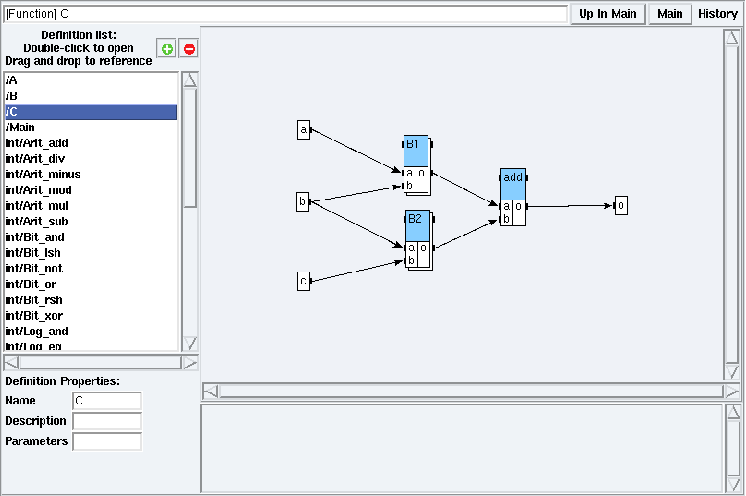Figure 5.4: C definition in examples/hierarchy/hierarchy.sdx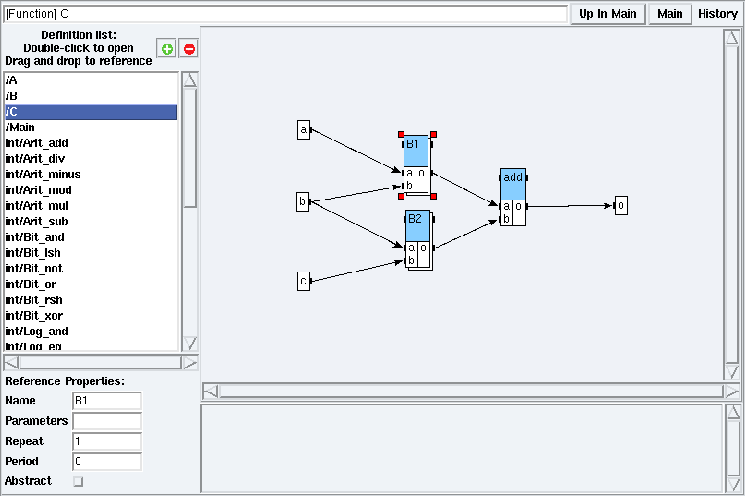Figure 5.5: Opening B1 reference in examples/hierarchy/hierarchy.sdx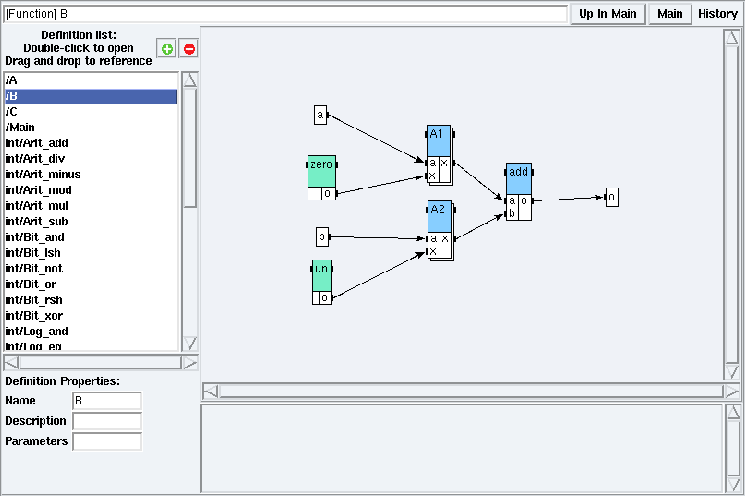Figure 5.6: B definition in examples/hierarchy/hierarchy.sdx

Double left click on a definition name in the Definition list (e.g. open the examples/hierarchy/hierarchy.sdx application and double left click on C in the Definition list). You are now in a definition mode (cf. figure 5.4). From a definition mode, to open the definition corresponding to a reference in order to inspect and possibly modify its content, left click on the target reference to select it. Red squares appear on its borders (cf. figure 5.5). Then double left click on it. It displays the definition of the target reference (cf. figure 5.6).

Note that as soon as you have included an algorithm library (cf. section 3.1), all its definitions appear in the definition list. The Definition list in figure 5.4 shows some local definitions (e.g. A, B, C, Main) and global definitions (e.g. int/Arit_add, int/Arit_div, etc.) since the integer library was included.

#### Main mode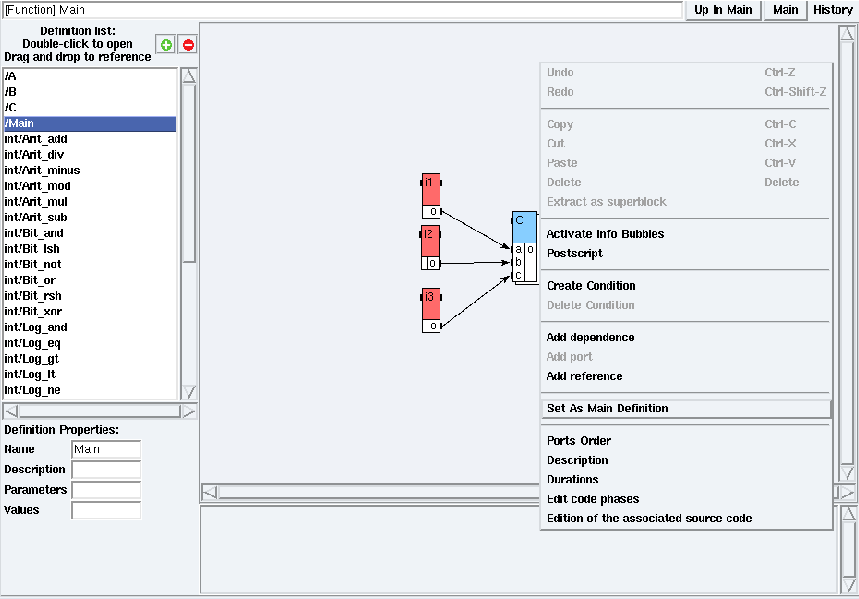Figure 5.7: Set Main definition as main algorithm in examples/hierarchy/hierarchy.sdx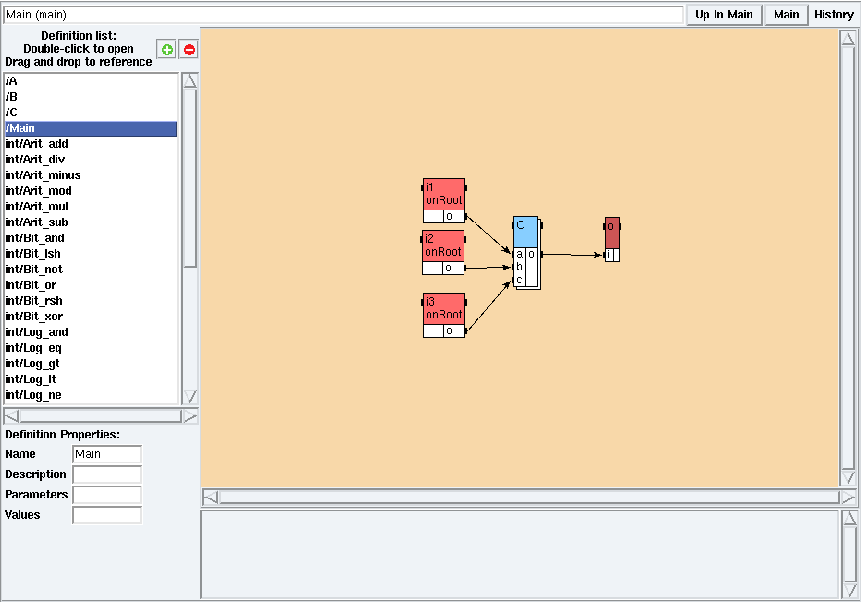Figure 5.8: Main mode in examples/hierarchy/hierarchy.sdx

To define an algorithm as main, right click on the background of the target definition window. Choose the Set As Main Definition option (cf. figure 5.7). The color of the background changes and the adress is changed from a [Function] to a (main), meaning that you are now in the main mode on the main algorithm (cf. figure 5.8). Note that the main algorithm must be at the root level of a hierarchy; it can not contain unconnected ports. Only the main algorithm can instanciate (give values to names) its parameters (cf. section 5.1.1) thanks to its field Values in its Definition Properties (cf. figure 5.8).
Left click on the Main button of the algorithm window. It displays the main algorithm in the main mode. Left click on a hierarchical reference to browse down the main algorithm (e.g. left click on the C reference of Main then left click on the B2 reference of C). Then left click on Up In Main to browse up the main algorithm.

#### Hierarchy

Now you may construct a graph with references to constants, sensors, actuators, delays and functions. If this definition is intended to be referenced in an explicit hierarchy, i.e. this reference will belong to a certain level of hierarchy (possibly a leaf), you must use input and output ports. If this definition is intended to be referenced at the root level of the hierarchy, input ports are replaced by sensors and output ports are replaced by actuators.
References to an explicitly hierarchical definition are displayed with a double-border (in the figure 5.4 B1 is a reference on an explicitly hierarchical definition contrary to add).

### 5.1.3  To create a port in a definition

Ports are communication interface of a definition with the outside world.

#### Direction of ports

SynDEx distinguishes three directions for ports:

• an input port represents a data that is provided by the outside world to the definition;
• an output port represents a data that is provided by the definition to the outside world;
• an input/output port can be seen as a reference (or pointer) to a data provided by the outside world that the definition can modify in place. This explains the name of input/output ports: we can read the value of the port and replace it by a new one.

#### New port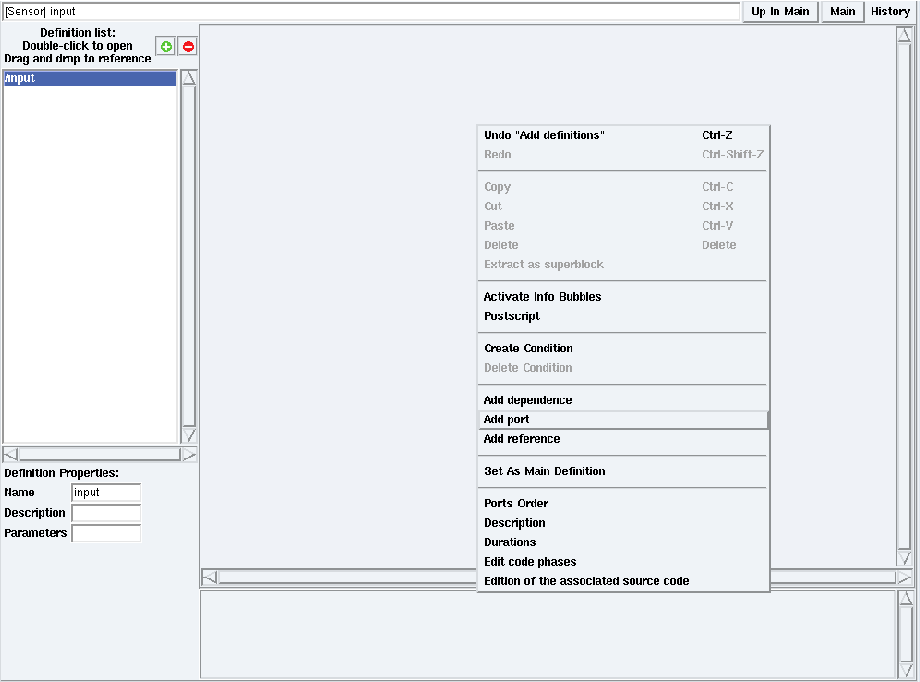Figure 5.9: Contextual menu → Create port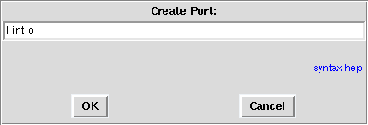Figure 5.10: Name of the new port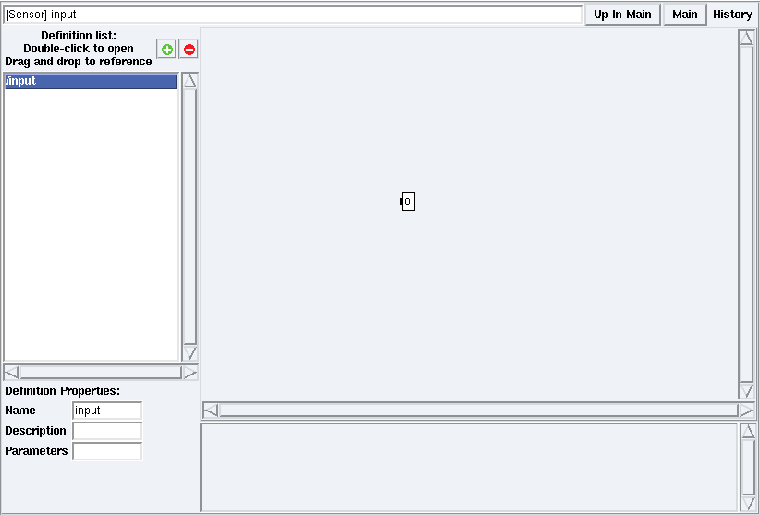Figure 5.11: A definition after port creation

To create a port in an atomic definition (cf. chapter 5):

• in the definition mode (cf. section 5.1.2), right click on the background and choose the Create port option For example create a new definition named input and create a port in this definition (cf. figure 5.9);
• it opens a dialog window in which you can type the port direction, type, name and optionally its size. You can left click on the syntax help link for more information. For example type ! int o, then left click OK (cf. figure 5.10);
• it creates the target port. In this example, the new port is an integer output port named o (cf. figure 5.11) in the definition window.

You can undo and redo this action, right click on the background and choose the Undo, Redo options.

A port definition has the following syntax:

```  port_definition ::= direction type [ "[" size "]" ] name
direction ::= "?" | "!" | "&"
```

where:

• ? specifies an input port,
• ! specifies an output port,
• & specifies an input/output port,

square brackets [...] represent optional elements, pipes ∣ represent alternatives, and keywords are quoted.
Hint: you can create several ports in one breath by simply putting several port definitions in a row in the dialog window, according to the following syntax:

```  port_definition ::= { port_definition }
```

where curly brackets {...} represent zero, one or several repetitions of the enclosed element.

#### Ports order

If you plan to generate code, it is necessary to specify an order for ports which is consistent with the declaration of the corresponding executable function. To specify the ports order, right click on the background and choose the Ports Order option.

#### Input/output ports

Input-output ports have a very specific behavior concerning data memory allocation in the executives generated by SynDEx. For any application, SynDEx makes data buffer allocations for (and only for) the output ports of the atomic references of your algorithm graph. Input-output ports do not cause an allocation but instead an alias on the output port of its predecessor. The operation containing this input-output port directly modifies the value of its predecessor port (side-effect). This is useful to avoid reallocation of big data buffers of the same type (for instances images) by making successive computations on the same data buffer.
However, as side-effects are not supposed to happen in data-flow graphs, this comes with some restrictions:

• Ports of delay definitions can not be input/output ports,
• Ports of hierarchical definitions can not be input/output ports,
• The data of an input/output port can not be diffused: if there is a dependence A.o --> B.io (where A.o is an output port and B.io is an input/output port), neither A.o nor B.io can be diffused (cf. section 5.3.1).

### 5.1.4  To create a reference in a definition

A reference can be thought as a call to a function in a traditional programming language. Here the called function is an algorithm definition.

#### New reference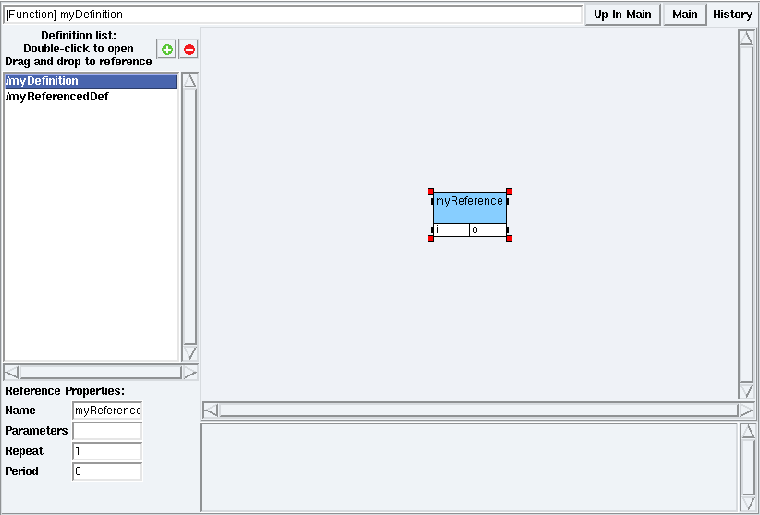Figure 5.12: A reference to myReferencedDef into myDefinition

To reference a definition (e.g. myReferencedDef) into another one (e.g. myDefinition), set the algorithm window in definition mode on myDefinition (cf. section 5.1.2). Then drag and drop myReferencedDef from the Definition list to the definition window (or select myReferencedDef in the Definition list, right click on the background of the definition window, and choose the Create reference option). It opens a dialog window. Type the name of the reference (e.g. myReference). See figure 5.12 to see the result.

#### Reference with parameters

To reference a definition with parameters (cf. section 5.1.1), a valued expression is required for each parameter of the definition. Parameters of a reference are valued expressions separated by semi-colon between < and > following the name of the reference, according to the syntax:

```  expr_list ::= "<" { expr ";" } expr ">"
expr ::= name | value | "(" expr ")" | expr "+" expr |
expr "-" expr | expr "*" expr | expr "/" expr |
"-" expr | "{" { expr "," } expr "}"
valued_expression ::= expr
```

where curly brackets {...} represent zero, one or several repetitions of the enclosed element, pipes ∣ represent alternatives, and keywords are quoted. A parameter is instanciated when it has a value otherwise it is not.

You can also edit the parameters in the Reference Properties with the same syntax. Note that the number of valued expressions must match the number of parameters of the referenced definition, and that types must match.

### 5.1.5  To create a dependence in a definition

A dependence is a directed edge connecting a producer operation to one or several consumer operations. As such, it specifies an execution order relation between two references used in a definition.

SynDEx distinguishes two types of dependences: data dependences and precedence dependences (without data) (cf. introduction of chapter 5). SynDEx automatically creates the right type of dependence depending on the context:

• To create a data dependence in a definition between two references, point the cursor at an output port (little black rectangle) of the source, middle click (or Ctrl left click), then drag and drop on an input port (little black rectangle) of the destination (or right click on the background, and choose the Add dependence option). The source and destination of a data dependence can also be ports: this is used to read a data from (resp. write a data to) the outside world. Note that for a given non-atomic definition, all output ports must be in dependence with input ports: all outputs must be defined;
• To create a precedence dependence in a definition between two references, point the cursor at an output precedence port (little black rectangle) of the source, middle click (or Ctrl left click), then drag and drop on an input precedence port (little black rectangle) of the destination. Input (resp. output) precedence ports are represented by little black squares at the left (resp.right) of the boxes holding the reference names.

### 5.1.6  To create a superblock

A superblock is a set of operations, edges and ports extracted as a new definition.

To create a definition as a superblock, select the target set of operations, edges and ports you want to extract (cf. section 4.1). Then right click and choose the Extract as superblock option. A new definition is created and a reference to this definition replaces the selected set. The new definition is available in the Definition list, You can rename both the definition and the reference.

You can undo and redo this action.

### 5.1.7  To create an abstract reference

An abstract reference is a reference to a hierarchical definition in which the hierarchy is not taken into account, i.e. the flattening (cf. section 9.5) does not go into the hierarchical referenced definition that becomes therefore abstract. However, note that to perform the adequation this definition must have a duration.

To create an abstract reference, select the desired hierarchical reference then, check the option Abstract in the Reference properties of this reference.

You can undo and redo this action.

## 5.2  To condition an algorithm definition

First make sure that the target definition contains an input port of type int for the conditioning port. Note that the SynDEx libs directory already provides an int library for operations on integer values.

#### New condition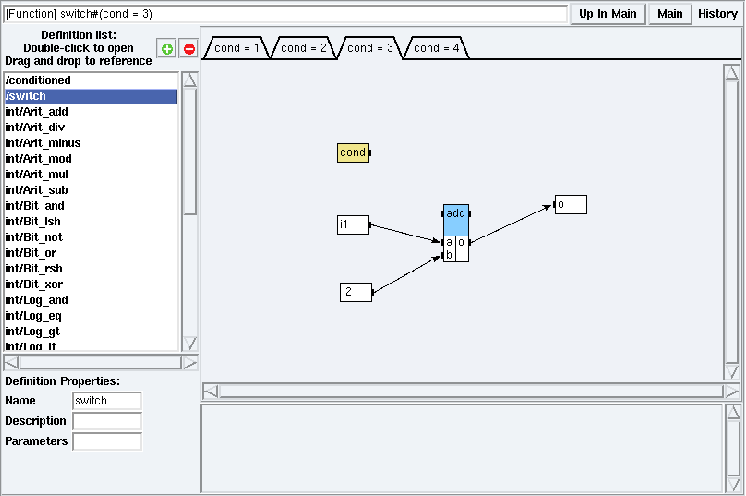Figure 5.13: switch definition mode for cond = 3 in examples/condition/simpleCondition/simpleCondition.sdx

Right click on the background of the definition window and choose the Create Condition option. It opens a dialog window for the new condition. A condition is a port = value expression where port is the name of the conditioning port and value is an integer. Note that the conditioning port must be of direction input (cf. 5.1.3). A new tab is created for the given condition. The conditioning port is now colored in yellow (cf. figure 5.13).

If necessary, refresh the algorithm window (cf. section 4.6).

#### Remarks

Note that there can be only one conditioning input port. You have to construct one sub-graph per value associated to a conditioning input port (cf. figure 5.13). For each other value of the conditioning input port, the result is unspecified and will be inconsistent.

#### CondI and CondO vertices

The adequation and the code generation will take into account the expanded graph (cf. section 9.5). SynDEx will introduce new vertices during the expansion: CondI and CondO vertices.

A CondI vertex consumes the conditioning data and connects the input ports of the conditioned operation according to its value.

A CondO vertex consumes the conditioning data and connects the output ports of the conditioned operation according to its value.

#### References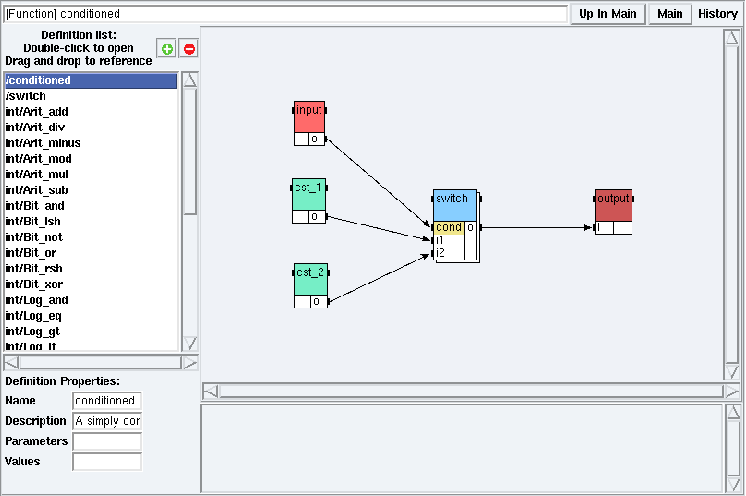Figure 5.14: conditioned definition mode in examples/condition/simpleCondition/simpleCondition.sdx

In a definition mode (cf. section 5.1.2), references to conditioned definitions have their conditioning port yellow colored (cf. figure 5.14).

#### Delete a condition

Right click on the background of the definition window and choose the Delete Condition option.

## 5.3  To repeat an algorithm definition

### 5.3.1  Diffuse, Fork, and Join

You can create a reference to a definition, and connect to its input (resp. output) ports some output (resp. input) ports with different sizes. The pre-condition is to have a unique common multiple between each pair of ports of different sizes. This multiple is the repetition factor of the reference.

#### Multiplication of a vector by a scalar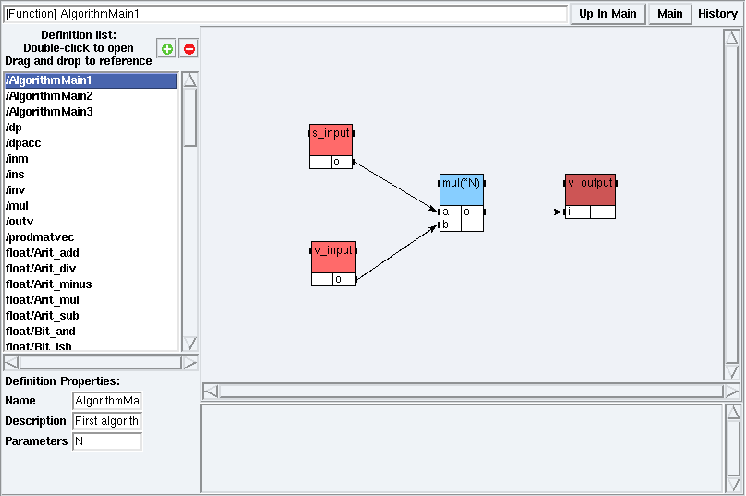Figure 5.15: AlgorithmMain1 definition mode in examples/tutorial/example4/example4.sdx

Suppose that you want to specify the multiplication of a vector by a scalar giving a vector as result (cf. AlgorithmMain1 in examples/tutorial/example4). You can specify it by repeating the multiplication between two scalars instead of defining a new one. For example for N length vectors, you may specify the repetition by N multiplications between scalars giving a scalar as a result (cf. figure 5.15).
You have to:

• create a definition with the parameter N,
• reference the multiplication on scalars mul,
• connect the output port of a scalar (e.g. s_input) to one of its input ports (e.g. mul.a),
• connect the output port of a vector (e.g. v_input) to the other input port (e.g. mul.b),
• connect its output port (mul.o) to the input port of a vector (e.g. v_output),
• set the repetition factor of mul to N: left click on the mul reference, then type N in its Reference Properties (cf. Algorithm window in chapter 5).

#### Repetition factor

The common multiple between each pair of ports with different sizes is N. It is the repetition factor that you have to set explicitely by using a symbolic numbered expression.

#### Diffuse the scalar

Since the output port of s_input has the same size as its connected input port of the multiplication function, it is replicated N times in order to be multiplicated by each element of v_input. This is a Diffuse operation.

#### Fork the vector

Since the function operates on scalars and the v_input vector has N elements, each of its elements are provided separately in order to be multiplicated. This is a Fork operation.

#### Join the internal results

Since the function operates on scalars and the v_output vector has N elements, each repetition of the multiplication is taken in order to be provided as a N elements vector. This is a Join operation.

#### Representation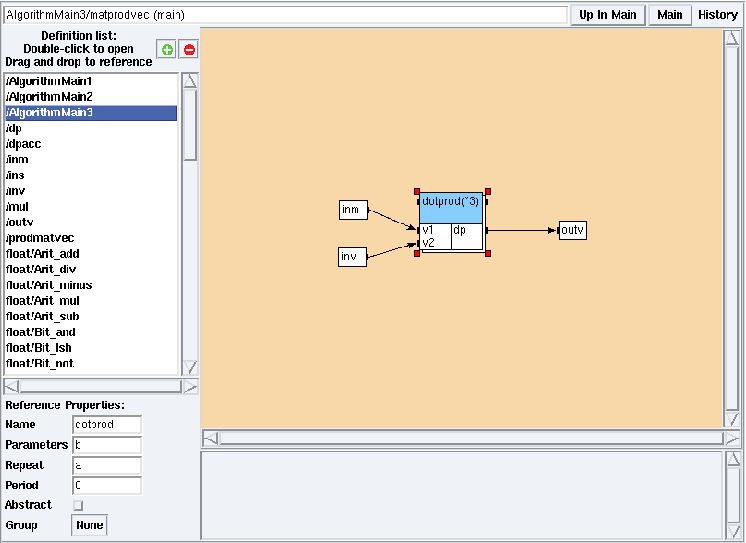Figure 5.16: matprodvec main mode from AlgorithmMain3 main algorithm in examples/tutorial/example4/example4.sdx

The repetition factor is displayed next to the name of the reference (e.g. in the figure 5.15 mul is repeated N times). The main algorithm (e.g. AlgorithmMain3) instanciates its parameters (cf. figure 5.8). From the main mode in examples/tutorial/example4/example4.sdx (cf. section 5.1.2), double left click on the matprodvec reference, the dotprod reference is repeated three times (cf. figure 5.16).

#### Explode and Implode vertices

The adequation and the code generation will take into account the expanded graph (cf. section 9.5). SynDEx will introduce new vertices during the expansion: Explode and Implode vertices.

An Explode vertex extracts for each repetition of a definition each element of the data it receives (cf. subsections Diffuse and Fork).

An Implode vertex builds the data it sends by concatenating each separated element produced by each repetition of the definition (cf. subsection Join).

### 5.3.2  Iterate

In some cases, you may want to repeat a reference but have no difference between port sizes.

#### Multiplication of two vectors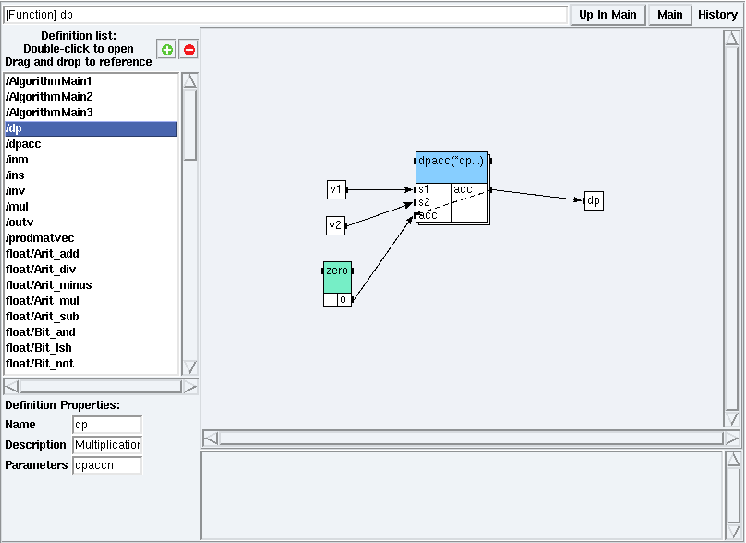Figure 5.17: dp definition mode in examples/tutorial/example4/example4.sdx

Suppose that you want to specify the multiplication of two vectors giving a scalar as a result (cf. figure 5.17). You can specify it by repeating the multiplication between two scalars, that used an accumulator to store the partial sum. For example if for dpaccn length vectors, you may specify the repetition by dpaccn multiplications between three scalars (the i element of the first vector, the i element of the second one, and the accumulator, initialized to 0).

You have to:

• reference the multiplication on scalars with accumulator (e.g. dp),
• connect two vectors (e.g. v1 and v2) to the scalar input ports of the multiplication,
• connect a {0} constant to the acc input port of the multiplication,
• connect the output port of the multiplication to a scalar (e.g. dp),
• connect the acc output port of the multiplication to its acc input port choosing an Iterate edge,
• repeat dpaccn times the multiplication (in the Reference Properties of the dpacc reference).

The accumulator is initialized with {0}. Then the output of the repetition i becomes the accumulator of the repetition i+1. The output of the last repetition is the output of the repeated definition. This is an Iterate operation.

## 5.4  To modify an algorithm definition or a reference

### 5.4.1  Modify a definition

Double left click on the definition name in the Definition List or double left click on a reference from a definition mode (cf. section 5.1.2). It opens its definition window. Right click on the background of the definition window. Choose the Create dependence option (cf. section 5.1.5), Create port (cf. section 5.1.3), Create reference (cf. section 5.1.4), Create Condition or Delete Condition (cf. section 5.2) to modify the definition.

As soon as you have Left clicked on the background of a definition window (cf. Algorithm window in chapter 5) you can change its Definition Properties to modify its Name, Description, Parameters or Values. The latter property appears only in the case of a main algorithm definition.

Note that you can modify local and global definitions (cf. section 3.1). Modifications on a global definition impact only the current application and the library remains unchanged. To modify a global definition over-all, open the corresponding SynDEx library file (e.g. libs/int.sdx). Modifications on a definition in a library may have consequences on all the applications using this library.

### 5.4.2  Modify a reference

Left click on a reference in a definition window (cf. Algorithm window in chapter 5). Use its Reference Properties to modify its Name, Parameters, Repeat or Period. For the period see the section 5.7 “To build mutli-periodic applications”.

## 5.5  To delete an algorithm definition

To delete a definition, in the algorithm window, left click on the - red button.

Note that deleting a global definition (cf. section 3.1) impacts only the current application.

## 5.6  To associate code with an algorithm definition

### 5.6.1  The code editor window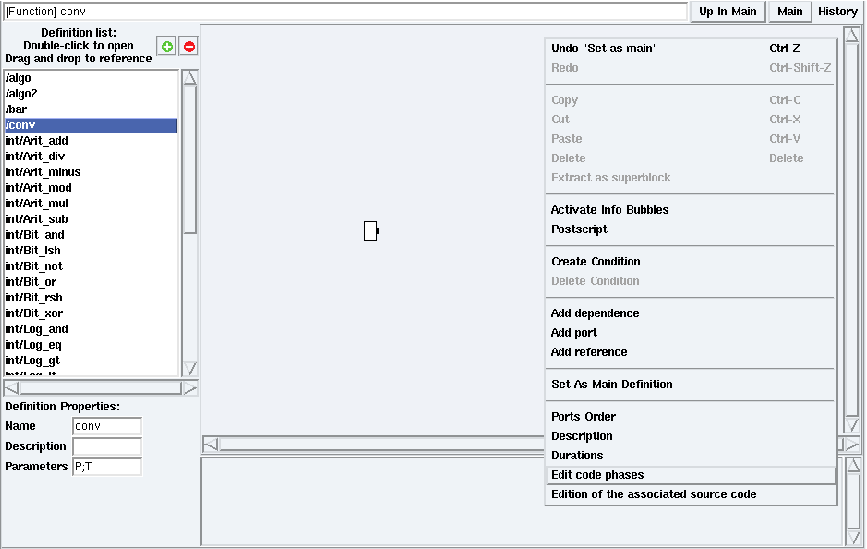Figure 5.18: Edition of the conv code phases in examples/tutorial/example7/example7.sdx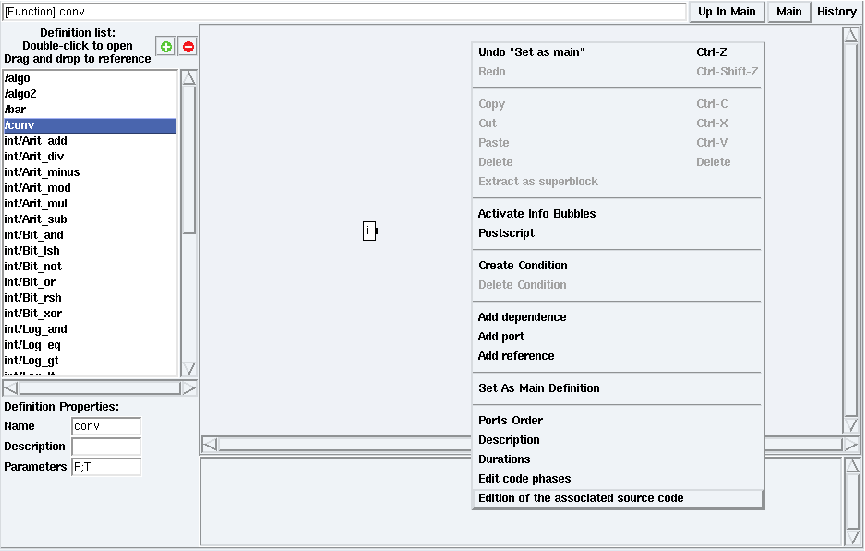Figure 5.19: Edition of the code associated with conv in examples/tutorial/example7/example7.sdx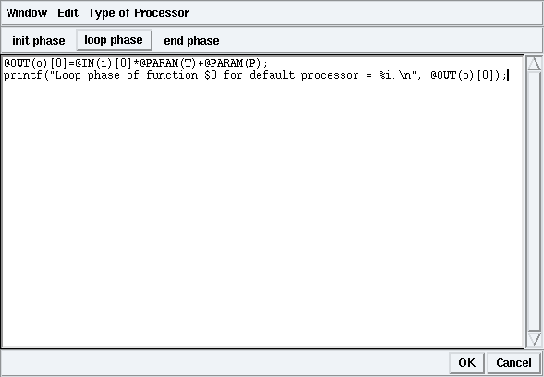Figure 5.20: Code associated with conv in loop phase in examples/tutorial/example7/example7.sdx

Right click on the background of a definition window. Choose the Edit code phases option (cf. figure 5.18). Check init (resp. end) to generate code in the initialization phase (resp. ending phase).

Right click on the background of a definition window. Choose the Edition of the associated source code option (cf. figure 5.19). It opens the code editor window on the initialization phase for the selected definition. Left click on loop phase (resp. end phase) to edit the code associated in the loop phase (resp. ending phase) (cf. figure 5.20).

### 5.6.2  The code editor macro language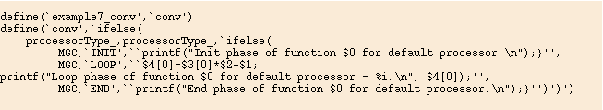Figure 5.21: M4 macro code for conv in examples/tutorial/example7/example7_sdc.sdx

From the Code menu of the principal window, check Generate m4x files. At code generation time, the code written in the code editor will be wrapped into M4 macro code, and outputed into an application_name_sdc.m4x file. These files contain one M4 macro definition per algorithm definition (cf. figure 5.21). The code editor offers several macros to abstract away the M4 nature of the output file. These macros are of two kinds: port and parameter names translation macros, and quoting macros (cf. macros directory).

#### Names translation macros

Parameter and port names of an algorithm definition are encoded as parameters of the corresponding M4 macro. Because the M4 language uses positional parameters, when you want to refer to a parameter or port in the associated code he has to know its position in the M4 macro parameters list. More than being not very handy, this is fragile relatively to creation or deletion of ports and parameters in the definition: when you create a port or a parameter to a definition, he has to adjust (replace \$n by \$n+1 in) all references to parameters or ports coming after the created one in the parameters list of the M4 macro. To overcome this difficulty, the code editor recognizes the following macros (cf. figure 5.20):

• @IN(prt) refers to the input port named prt,
• @OUT(prt) refers to the output port named prt,
• @INOUT(prt) refers to the input/output port named prt,
• @PARAM(prm) refers to the parameter named prm,
• @NAME(pr) refers to the port or parameter named pr. When using this macro, you should be careful that the port or parameter you want to refer to has a unique name in the definition.

#### Quoting macros

Quoting macros are used to wrap or unwrap code by M4 quote. The code editor recognizes the following quoting macros:

• @QUOTE(txt) will be put as ‘txt’ in the output file,
• @TEXT(‘txt’) will be put as txt in the output file.

### 5.6.3  The code editor shortcuts

The code editor supports various keyboard shortcuts that could be handy when editing source code.

 Ctr-Tab Insert a tabulation. Tab Complete a port name. Type the beginning of a port name, then press Tab and as many times as necessary for the editor to find the wanted completion. Ctr-I Insert the @IN macro at cursor position. Ctr-O Insert the @OUT macro at cursor position. Ctr-N Insert the @INOUT macro at cursor position. Ctr-P Insert the @PARAM macro at cursor position. Ctr-T Insert the @TEXT macro at cursor position. Ctr-Q Insert the @QUOTE macro at cursor position. Ctr-W Cut the selected text into the clipboard. Ctr-K Cut text from cursor position to the end of the line. Alt-W Copy the selected text into the clipboard. Ctr-Y Paste the clipboard content at cursor position. Ctr-A Jump to the beginning of the line. Ctr-E Jump to the end of the line. Ctr-up Jump to the beginning of the buffer. Ctr-down Jump to the end of the buffer.

## 5.7  To build multi-periodic applications

Until version 6 of SynDEx a unique timing information (execution duration) is associated to each operation (resp. each data type of a dependence) relatively to the operators (resp. media) it may be distributed onto. This timing information, which depends on the hardware, is associated to the definition of every operation. Applications specified by the user with version 6 are implicitely mono-periodic, meaning that all the operations of the algorithm graph have the same period which is equal to the total execution time of all the operations executed on the different components of the architecture, taking into account the duration of data communications through the media. This total execution time is displayed as the value of the “Cycle time” in the schedule window resulting from the adequation.

Version 7 of SynDEx allows the user to specify, in addition to a duration, a period to each operation. The period is a timing information associated to the reference of an operation instead of its definition, which does not depend on the hardware. This feature allows the user to specify an operation definition with the same execution duration each time it is referenced, whereas this operation may be referenced with several periods. Note that for a given operation it is necessary that its execution duration is smaller than its period to be schedulable.

As soon as a period is associated to an operation reference, the application becomes multi-periodic, and a period must be associated to every operation reference. If it is not the case the application remains mono-periodic. For both mono-periodic and multi-periodic applications, execution durations must be associated to operation definitions and data type of dependences. A multi-periodic application has a hyper period equal to the LCM (Least Common Multiple) of all the periods associated to the operation references. This hyper period is displayed as the value of the “Cycle time” in the schedule window resulting from the adequation. Note that the “Cycle time” is different from the total execution time of all the operations executed on the different components of the architecture, taking into account the duration of data communications through the media.

Version 7 of SynDEx, using the period and the execution duration of every operation, performs a distributed real-time schedulability analysis. If the application is schedulable, SynDEx may generate the corresponding macro-code (or may not find any schedule).

Version 7 of SynDEx, using the period and the execution duration of every operation, performs a distributed real-time schedulability analysis to determine if the multi-periodic application is schedulable. If it is the case it will generate the corresponding macro-code.

#### Multiple or equal periods

Operations related by a dependence must have multiple or equal periods. While creating a dependence between operations which have inconsistent periods, an error message appears to help the user (e.g. Can not create dependence input.o -> compute.in in definition basicAlgorithm Error #1 [Inconsistent periods]).

While creating a dependence between operations which have multiple periods, there are two cases:

• the producer operation has a period p smaller than the period n of the consumer operation. In this case the producer operation executes n/p times more than the consumer operation and consequently, produces n/p data for the consumer operation involving that these data are memorized. SynDEx displays a warning message indicating that the destination port’s size will be increased (e.g. #1 Warning about dependence input.o -> compute.in in definition basicAlgorithm [The size of destination compute.in will increase to 2 times the original size]). In addition, it creates a new operation called with the data type of the dependence prefixed by “Implode_” (e.g. Implode_int). This new operation is in charge of collecting the n/p data for the consumer operation. Note that the user must give a duration to this new operation. In case he forgot it a warning message will ask for during the adequation ;
• the producer operation has a period p greater than the period n of the consumer operation. In this case the consumer operation executes p/n times more than the producer operation and consequently, the consumer operation consumes p/n times the same data.

#### Hierarchical references

Verifications on periods are propagated to hierarchical references.

While setting the period to a hierarchical reference, SynDEx verifies that the new period is compatible with the periods of the references it contains. Actually, the period of a hierarchical reference must be equal (or multiple) to the Least Common Multiple (LCM) to the periods of the references it contains.

While setting the period to a reference contained in a hierarchical reference, SynDEx verifies that the new period is compatible with the period of the hierarchical reference. Actually, the period of a reference contained in a hierarchical reference must be equal (or must be a divisor) to the period of the hierarchical reference.

#### Edit the period of an operation

The user can edit the period of an operation only in its reference properties (cf. paragraph “Algorithm window” in section 5) unlike its name, its parameters and its repeat factor which can also be edited during the reference creation.

By default the period of an operation is equal to 0. Note that, as soon as an operation has a period equal to 0, the application is mono-periodic whatever the other periods are. In other words, to obtain a multi-periodic application the period of all the references must be edited.